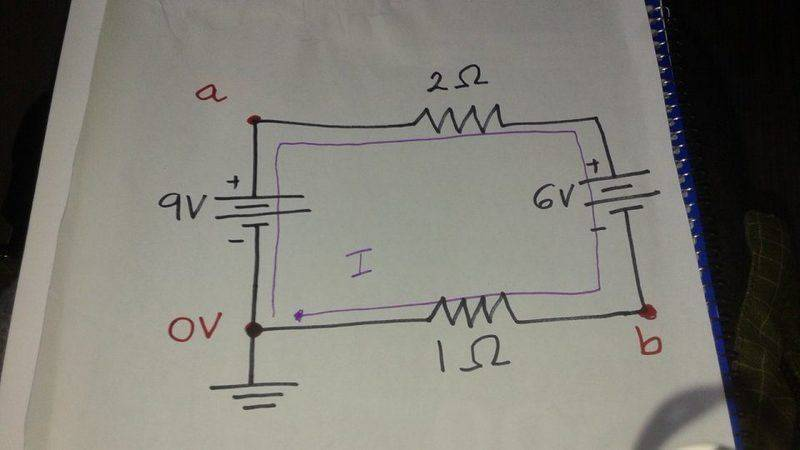# Grounded circuit, 2 batteries and 2 resistors

kamhogo

## Homework Statement

Picture a circuit grounded at the left lower corner. Then as you go up the left side of the circuit, you have a 9V battery. The top left corner is labeled a. Then on the top of the circuit, you have a 2 ohms resistor. Then on the right side of the circuit, you have a 6V battery. The right lower corner is labeled b. Then on the bottom side of the circuit you have a 1 ohm resistor.
What is the value of the potential at points a and b?

## Homework Equations

Kirchhoff's loop law
I = Epsilon/R
Delta Vr = - I*R

## The Attempt at a Solution

First Kirchhoff's loop law to find the current in the circuit. I assumed the clockwise current was the same in both resistors.

--> 9V - 2 I - 6V - 1 I = 0
3V = 3I --> I = 1A

I know the potential at point a is 9V. The potential at the lower left corner, where the circuit is grounded, is zero. As you go up the circuit, you have gained 9 V by "passing through" the 9V battery.

Then you pass through the top resistor.
The potential difference across that resistor is given by delta Vr = - I * R = -1A*2omhs =-2V. So by the time you reach the right top corner, you are at 9V-2V= 7V.

Then you pass through the 6V battery. You go from the positive terminal to the negative terminal, so you lose 6V. Hence, at point b you are 7V-6V= 1V.

Is my reasoning any good?

## Answers and Replies

Homework Helper
Gold Member
Then as you go up the left side of the circuit, you have a 9V battery. The top left corner is labeled a. Then on the top of the circuit, you have a 2 ohms resistor. Then on the right side of the circuit, you have a 6V battery. The right lower corner is labeled b. Then on the bottom side of the circuit you have a 1 ohm resistor.
What is the value of the potential at points a and b?
What about polaritiy of the batteries? A diagram would be helpful.

kamhogoHomework Helper
Gold Member
Is my reasoning any good?
Yes, that all works.

kamhogo
Yes, that all works.

Muchas gracias! !

Homework Helper
Gold Member
You can also get to b by going "up" through the 1 Ohm....

0 + (1*1) = 1V

kamhogo
You can also get to b by going "up" through the 1 Ohm....

0 + (1*1) = 1V

True that.